# Work with Prometheus counters

Use Flux to query and transform Prometheus counter metrics stored in InfluxDB.

A counter is a cumulative metric that represents a single monotonically increasing counter whose value can only increase or be reset to zero on restart.

Prometheus metric types

##### Example counter metric in Prometheus format
``````# HELP example_counter_total Total representing an example counter metric
# TYPE example_counter_total counter
example_counter_total 282327
``````

Because counters can periodically reset to 0, any query involving counter metrics should normalize the data to account for counter resets before further processing.

The examples below include example data collected from the InfluxDB OSS 2.x `/metrics` endpoint using `prometheus.scrape()` and stored in InfluxDB.

#### Prometheus metric parsing formats

Query structure depends on the Prometheus metric parsing format used to scrape the Prometheus metrics. Select the appropriate metric format version below.

## Normalize counter resets

1. Filter results by the `prometheus` measurement and counter metric name field.
2. Use `increase()` to normalize counter resets. `increase()` returns the cumulative sum of positive changes in column values.

`increase()` accounts for counter resets, but may lose some precision on reset depending on your scrape interval. On counter reset, `increase()` assumes no increase.

``````from(bucket: "example-bucket")
|> range(start: -1m)
|> filter(fn: (r) => r._measurement == "prometheus" and r._field == "http_query_request_bytes")
|> increase()
``````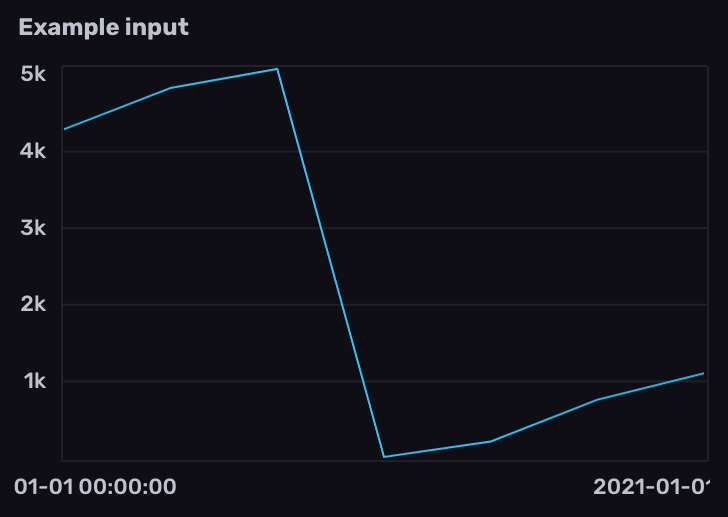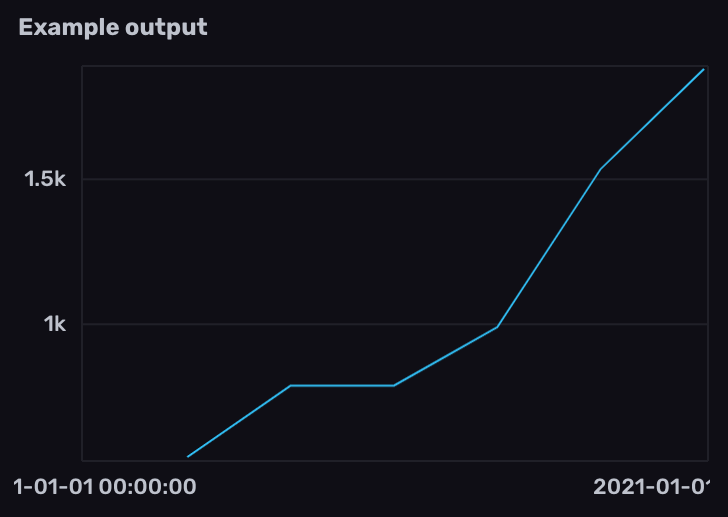View example input and output data

1. Filter results by the counter metric name measurement and `counter` field.
2. Use `increase()` to normalize counter resets. `increase()` returns the cumulative sum of positive changes in column values.

`increase()` accounts for counter resets, but may lose some precision on reset depending on your scrape interval. On counter reset, `increase()` assumes no increase.

``````from(bucket: "example-bucket")
|> range(start: -1m)
|> filter(fn: (r) => r._measurement == "http_query_request_bytes" and r._field == "counter")
|> increase()
``````View example input and output data

## Calculate changes between normalized counter values

Use `difference()` with normalized counter data to return the difference between subsequent values.

``````from(bucket: "example-bucket")
|> range(start: -1m)
|> filter(fn: (r) => r._measurement == "prometheus" and r._field == "http_query_request_bytes")
|> increase()
|> difference()
``````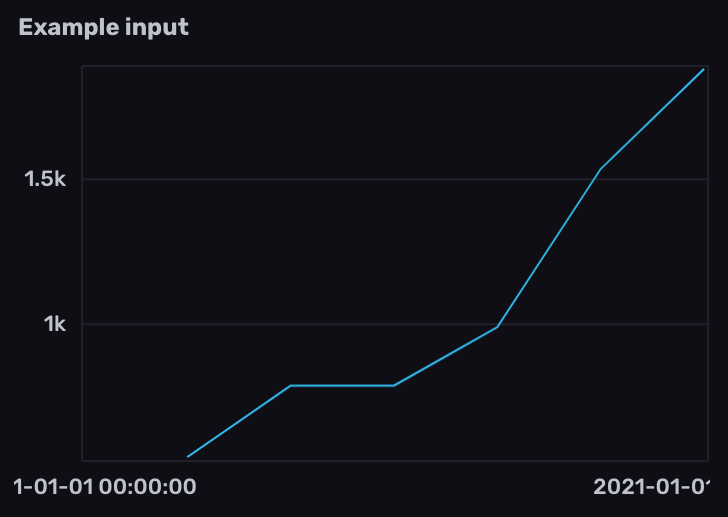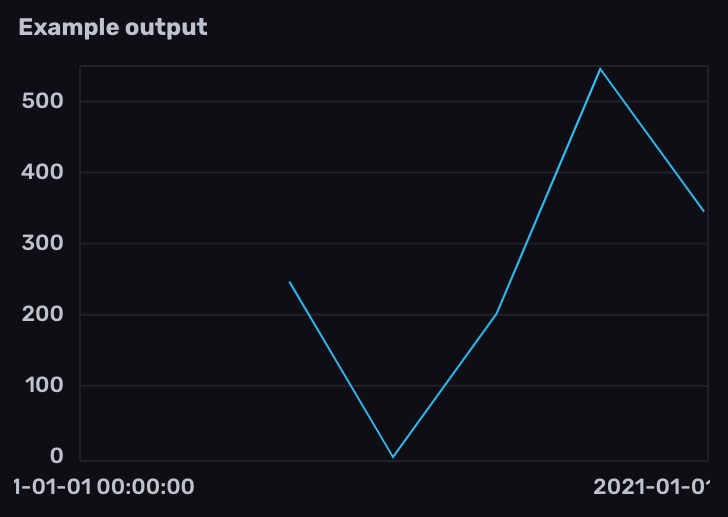View example input and output data

``````from(bucket: "example-bucket")
|> range(start: -1m)
|> filter(fn: (r) => r._measurement == "http_query_request_bytes" and r._field == "counter")
|> increase()
|> difference()
``````View example input and output data

## Calculate the rate of change in normalized counter values

Use `derivative()` to calculate the rate of change between normalized counter values. By default, `derivative()` returns the rate of change per second. Use the `unit` parameter to customize the rate unit.

``````from(bucket: "example-bucket")
|> range(start: -1m)
|> filter(fn: (r) => r._measurement == "prometheus" and r._field == "http_query_request_bytes")
|> increase()
|> derivative()
``````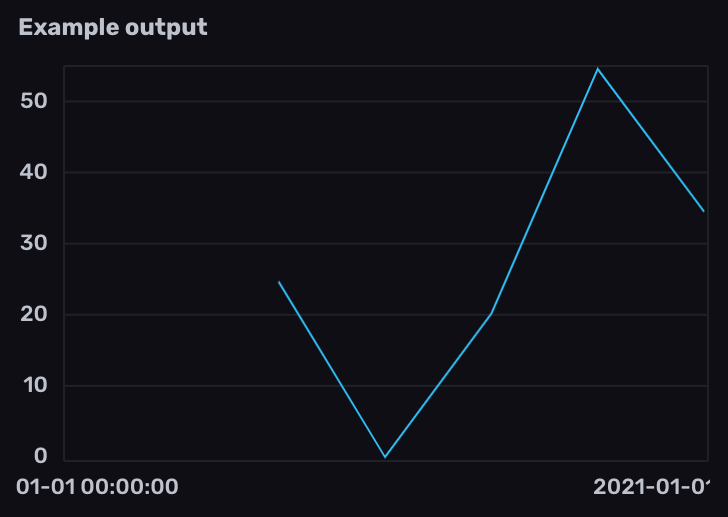View example input and output data

``````from(bucket: "example-bucket")
|> range(start: -1m)
|> filter(fn: (r) => r._measurement == "http_query_request_bytes" and r._field == "counter")
|> increase()
|> derivative()
``````View example input and output data

## Calculate the average rate of change in specified time windows

To calculate the average rate of change in normalized counter values in specified time windows:

1. Import the `experimental/aggregate` package.

2. Use `aggregate.rate()` to calculate the average rate of change per time window.

``````import "experimental/aggregate"

from(bucket: "example-bucket")
|> range(start: -1m)
|> filter(fn: (r) => r._measurement == "prometheus" and r._field == "http_query_request_bytes")
|> increase()
|> aggregate.rate(every: 15s, unit: 1s)
``````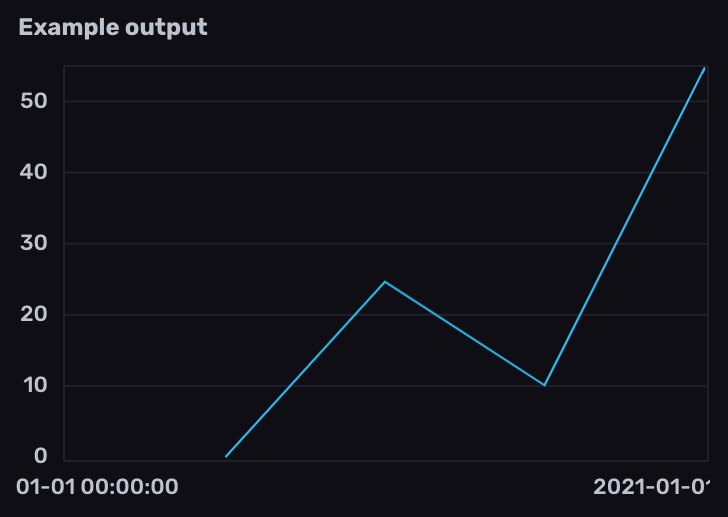View example input and output data

``````import "experimental/aggregate"

from(bucket: "example-bucket")
|> range(start: -1m)
|> filter(fn: (r) => r._measurement == "http_query_request_bytes" and r._field == "counter")
|> increase()
|> aggregate.rate(every: 15s, unit: 1s)
``````View example input and output data